# Math's project.pptx

May. 28, 2023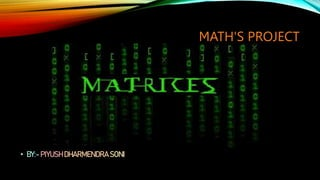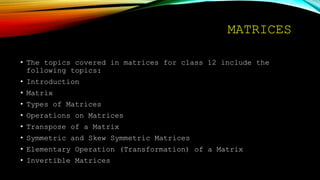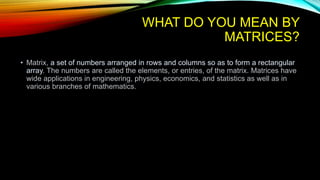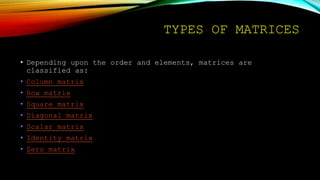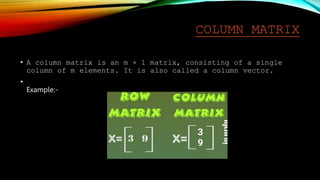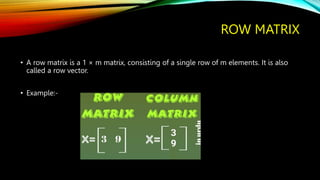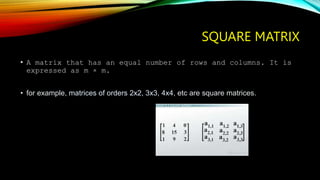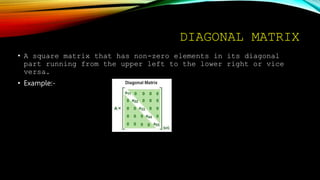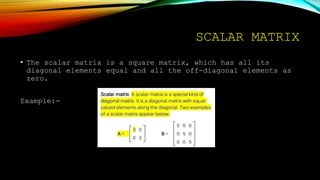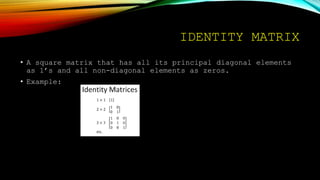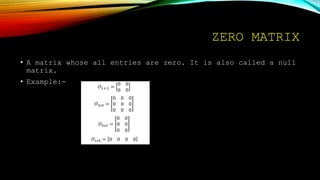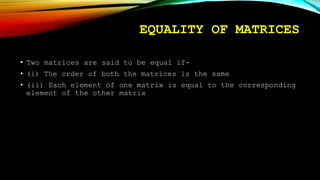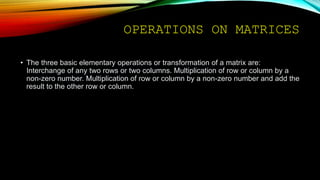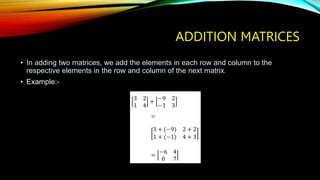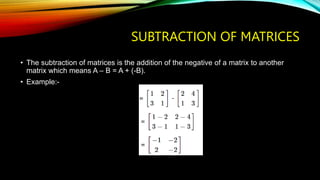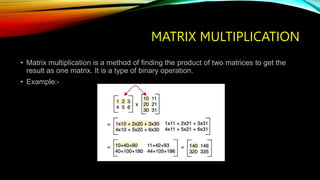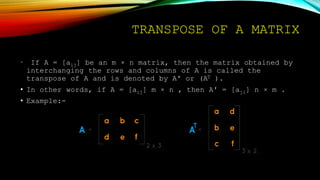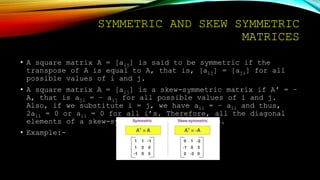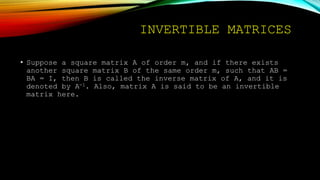1 of 21

### Math's project.pptx

• 1. MATH'S PROJECT • BY:- PIYUSH DHARMENDRA SONI
• 2. MATRICES A matrix is a function that consists of an ordered rectangular array of numbers. The numbers in the array are called the entities or the elements of the matrix. The horizontal array of elements in the matrix is called rows, and the vertical array of elements are called the columns.
• 3. MATRICES • The topics covered in matrices for class 12 include the following topics: • Introduction • Matrix • Types of Matrices • Operations on Matrices • Transpose of a Matrix • Symmetric and Skew Symmetric Matrices • Elementary Operation (Transformation) of a Matrix • Invertible Matrices
• 4. WHAT DO YOU MEAN BY MATRICES? • Matrix, a set of numbers arranged in rows and columns so as to form a rectangular array. The numbers are called the elements, or entries, of the matrix. Matrices have wide applications in engineering, physics, economics, and statistics as well as in various branches of mathematics.
• 5. TYPES OF MATRICES • Depending upon the order and elements, matrices are classified as: • Column matrix • Row matrix • Square matrix • Diagonal matrix • Scalar matrix • Identity matrix • Zero matrix
• 6. COLUMN MATRIX • A column matrix is an m × 1 matrix, consisting of a single column of m elements. It is also called a column vector. • Example:-
• 7. ROW MATRIX • A row matrix is a 1 × m matrix, consisting of a single row of m elements. It is also called a row vector. • Example:-
• 8. SQUARE MATRIX • A matrix that has an equal number of rows and columns. It is expressed as m × m. • for example, matrices of orders 2x2, 3x3, 4x4, etc are square matrices.
• 9. DIAGONAL MATRIX • A square matrix that has non-zero elements in its diagonal part running from the upper left to the lower right or vice versa. • Example:-
• 10. SCALAR MATRIX • The scalar matrix is a square matrix, which has all its diagonal elements equal and all the off-diagonal elements as zero. Example:-
• 11. IDENTITY MATRIX • A square matrix that has all its principal diagonal elements as 1’s and all non-diagonal elements as zeros. • Example:
• 12. ZERO MATRIX • A matrix whose all entries are zero. It is also called a null matrix. • Example:-
• 13. EQUALITY OF MATRICES • Two matrices are said to be equal if- • (i) The order of both the matrices is the same • (ii) Each element of one matrix is equal to the corresponding element of the other matrix
• 14. OPERATIONS ON MATRICES • The three basic elementary operations or transformation of a matrix are: Interchange of any two rows or two columns. Multiplication of row or column by a non-zero number. Multiplication of row or column by a non-zero number and add the result to the other row or column.
• 15. ADDITION MATRICES • In adding two matrices, we add the elements in each row and column to the respective elements in the row and column of the next matrix. • Example:-
• 16. SUBTRACTION OF MATRICES • The subtraction of matrices is the addition of the negative of a matrix to another matrix which means A – B = A + (-B). • Example:-
• 17. MATRIX MULTIPLICATION • Matrix multiplication is a method of finding the product of two matrices to get the result as one matrix. It is a type of binary operation. • Example:-
• 18. TRANSPOSE OF A MATRIX • If A = [aij] be an m × n matrix, then the matrix obtained by interchanging the rows and columns of A is called the transpose of A and is denoted by A′ or (AT ). • In other words, if A = [aij] m × n , then A′ = [aji] n × m . • Example:-
• 19. SYMMETRIC AND SKEW SYMMETRIC MATRICES • A square matrix A = [aij] is said to be symmetric if the transpose of A is equal to A, that is, [aij] = [aji] for all possible values of i and j. • A square matrix A = [aij] is a skew-symmetric matrix if A′ = – A, that is aji = – aij for all possible values of i and j. Also, if we substitute i = j, we have aii = – aii and thus, 2aii = 0 or aii = 0 for all i’s. Therefore, all the diagonal elements of a skew-symmetric matrix are zero. • Example:-
• 20. INVERTIBLE MATRICES • Suppose a square matrix A of order m, and if there exists another square matrix B of the same order m, such that AB = BA = I, then B is called the inverse matrix of A, and it is denoted by A-1. Also, matrix A is said to be an invertible matrix here.
• 21. THANK YOU!! •